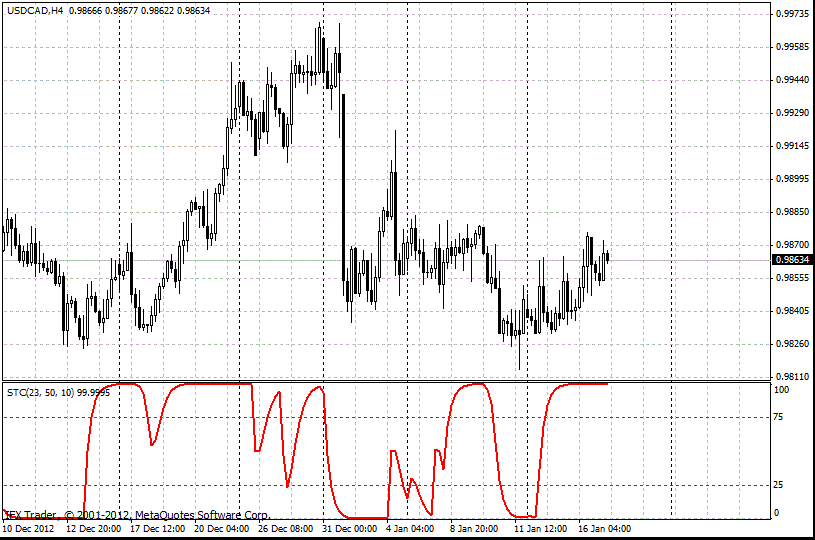Schaff Trend Cycle Indicator

# Schaff Trend Cycle Indicator

The Schaff Trend Cycle Indicator (STC) was developed by Doug Schaff in 2008. It is based on the assumption that currency trends accelerate and slow down in a cyclical pattern.

#### Formula

1. Moving averages (23- and 50-period EMA) and the MACD values are calculated.

EMA1 = EMA(Close, ShortLength);

EMA2 = EMA(Clost, LongLength);

MACD = EMA1 – EMA2;

2. 10-period Stochastic from the MACD values are calculated (cycles of the Schaff Trend Cycle).

%K(MACD) = %KV(MACD, 10);

%D(MACD) = %DV(MACD ,10);

Schaff = 100 * (MACD – %K(MACD)) / (%D(MACD) – %K(MACD));

The technical indicator STC is oscillator from the technical point of view. It is based on the principles of the Stochastic Oscillator and the MACD. The STC detects up and down trends, ignoring a lot of short-term periods of noise.

According to the concept of the STC Indicator, after a particular time on the Forex market, the trend returns to a starting point of its development, a cycle of its further movements starts to repeat. Thus, some kind of cyclic recurrence occurs in price fluctuations in the Forex market. It is detected by the STC Indicator for metatrader.

During the process of the Index’s development, besides cyclicity, combination of two different approaches to the evaluation of trend’s movement was used: the smoothed stochastic oscillator and the MACD.

The STC Indicator functions the following way. It generates its buy signal when the signal line turns up from 25 to indicate a long or turns down from 75 to indicate a short.

The developer of the indicator suggested the following method of signal filtering. If a bar, which is followed by a signal bar, closes higher than the high of the previous one, then there is a great possibility of price movements, and as a consequence to buy. On the contrary, when a ban, which is followed by a signal bar, closes lower than the low of the previous bar, there is signal to sell. It presupposes the high probability of profitable sells.#### Parameters of the InstaForex Schaff Trend Cycle

MAshort = 23

MAlong = 50

Cycle = 10

Can't speak right now?